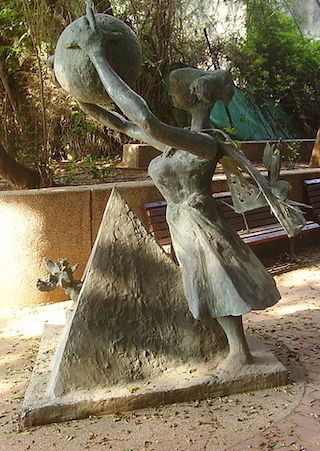# The Largest Sphere Within A Pyramid

Geometry Level 5$ABCD$ is a convex quadrilateral such that $[ABD] = [CDB]$, $|AB| = 1$ and $|BC|=|CD|$. $S$ is a point in space such that $|AS| + |DS| = \sqrt{2}$ and the volume of the pyramid $SABCD$ is equal to $\frac{1}{6}$.

The surface area of the largest ball that can fit inside such a pyramid can be expressed as $\frac{a-\sqrt{b}}{c} \pi ,$ where $a,b,c$ are positive integers, with $c$ the smallest possible. What is $a+b+c?$

×